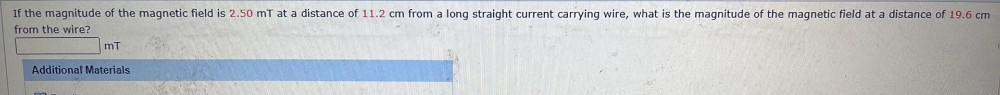Question:

# If the magnitude of the magnetic field is 2.50 mt at a distance of 11.2 cm from a long straight current carrying wire, what is tIf the magnitude of the magnetic field is 2.50 mt at a distance of 11.2 cm from a long straight current carrying wire, what is the magnitude of the magnetic field at a distance of 19.6 cm from the wire? mt Additional Materials